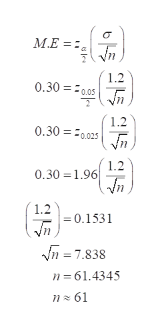# A research group wishes to estimate the mean number of hours that high school students spend watching TV on a weekday.A margin of error ofe=0.30 hour is desired. Past studies suggest that a population standard deviation ofσ=1.2 hours is reasonable. Estimate the minimum sample size required to estimate the population mean with​ 95% confidence.The required sample size is__________?​(Round up to the nearest whole​ number.)

Question
4 views
A research group wishes to estimate the mean number of hours that high school students spend watching TV on a weekday.
A margin of error of
e=0.30 hour is desired. Past studies suggest that a population standard deviation of
σ=1.2 hours is reasonable. Estimate the minimum sample size required to estimate the population mean with​ 95% confidence.
The required sample size is
__________?
​(Round up to the nearest whole​ number.)
check_circle

Step 1

Given that,

Margin of error = 0.30

Population standard deviation σ =1.2

Confidence interval 95%.

Sample size=?

Step 2

The formula for calculating sample size is

Step 3

Sub the given ...help_outlineImage Transcriptioncloseм. M.E =: 1.2 0,30 =0.05Jn 1.2 0.30 = 0.025 1.2 0.30 = 1.96 1.2 = 0.1531 n = 7.838 n = 61.4345 nz 61 fullscreen

### Want to see the full answer?

See Solution

#### Want to see this answer and more?

Solutions are written by subject experts who are available 24/7. Questions are typically answered within 1 hour.*

See Solution
*Response times may vary by subject and question.
Tagged in

### Statistics